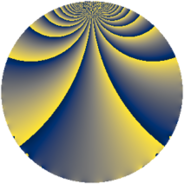# Properties

 Label 920.2.jLevel $920$ Weight $2$ Character orbit 920.j Rep. character $\chi_{920}(829,\cdot)$ Character field $\Q$ Dimension $132$ Newform subspaces $1$ Sturm bound $288$ Trace bound $0$

# Related objects

## Defining parameters

 Level: $$N$$ $$=$$ $$920 = 2^{3} \cdot 5 \cdot 23$$ Weight: $$k$$ $$=$$ $$2$$ Character orbit: $$[\chi]$$ $$=$$ 920.j (of order $$2$$ and degree $$1$$) Character conductor: $$\operatorname{cond}(\chi)$$ $$=$$ $$40$$ Character field: $$\Q$$ Newform subspaces: $$1$$ Sturm bound: $$288$$ Trace bound: $$0$$

## Dimensions

The following table gives the dimensions of various subspaces of $$M_{2}(920, [\chi])$$.

Total New Old
Modular forms 148 132 16
Cusp forms 140 132 8
Eisenstein series 8 0 8

## Trace form

 $$132q + 132q^{9} + O(q^{10})$$ $$132q + 132q^{9} - 14q^{10} - 20q^{14} + 8q^{16} - 8q^{20} - 44q^{24} + 4q^{25} + 8q^{26} + 14q^{30} - 24q^{31} + 8q^{34} - 56q^{36} - 32q^{39} + 6q^{40} - 8q^{41} + 36q^{44} - 132q^{49} - 12q^{50} - 12q^{54} - 32q^{55} - 36q^{56} - 48q^{60} - 60q^{64} - 24q^{65} - 32q^{66} + 28q^{70} + 88q^{71} + 44q^{74} - 84q^{76} + 54q^{80} + 132q^{81} + 108q^{84} - 68q^{86} - 40q^{89} + 20q^{90} - 12q^{94} + 24q^{95} + 24q^{96} + O(q^{100})$$

## Decomposition of $$S_{2}^{\mathrm{new}}(920, [\chi])$$ into newform subspaces

Label Dim. $$A$$ Field CM Traces $q$-expansion
$$a_2$$ $$a_3$$ $$a_5$$ $$a_7$$
920.2.j.a $$132$$ $$7.346$$ None $$0$$ $$0$$ $$0$$ $$0$$

## Decomposition of $$S_{2}^{\mathrm{old}}(920, [\chi])$$ into lower level spaces

$$S_{2}^{\mathrm{old}}(920, [\chi]) \cong$$ $$S_{2}^{\mathrm{new}}(40, [\chi])$$$$^{\oplus 2}$$